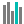#FINV (PQL - xl)

This function returns the inverse of the F probability distribution

• Library: PQL \ Spreadsheet \ Statistical
• Compatibility: Any content (regardless of data source) in the Tabulate spreadsheet module
• Solve: This function can be used with the Solve plug-in: both as part of the objective function and constraint functions

#### Syntax

FINV(probability, deg freedom1, deg freedom2)

##### Function Arguments
 Name Description Type Optional probability Probability associated with the F cumulative distribution Number deg freedom1 Number of degrees of freedom for the numerator; if not an integer, the number is truncated Number deg freedom2 Number of degrees of freedom for the denominator; if not an integer, the number is truncated Number